Next: Normalization scheme Up: Particle-in-cell codes Previous: Particle-in-cell codes

## Introduction

Consider an unmagnetized, uniform, 1-dimensional plasma consisting ofelectrons andunit-charged ions. Now, ions are much more massive than electrons. Hence, on short time-scales, we can treat the ions as a static neutralizing background, and only consider the motion of the electrons. Let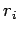be the-coordinate of the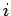th electron. The equations of motion of theth electron are written: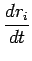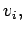(289)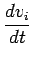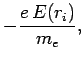(290)

where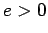is the magnitude of the electron charge,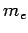the electron mass, and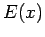the-component of the electric field-strength at position. Now, the electric field-strength can be expressed in terms of an electric potential: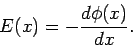(291)

Furthermore, from the Poisson-Maxwell equation, we have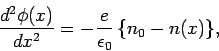(292)

where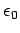is the permittivity of free-space,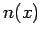the electron number density (i.e.,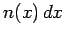is the number of electrons in the intervalto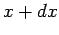), and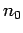the uniform ion number density. Of course, the average value ofis equal to, since there are equal numbers of ions and electrons.

Let us consider an initial electron distribution function consisting of two counter-propagating Maxwellian beams of mean speed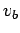and thermal spread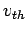: i.e.,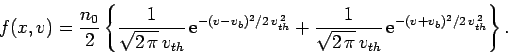(293)

Here,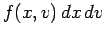is the number of electrons betweenandwith velocities in the rangeto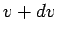. Of course,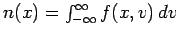. The beam temperature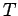is related to the thermal velocity via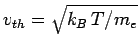, where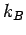is the Boltzmann constant. It is well-known that ifis significantly larger thanthen the above distribution is unstable to a plasma instability called the two-stream instability.38 Let us investigate this instability numerically.Next: Normalization scheme Up: Particle-in-cell codes Previous: Particle-in-cell codes
Richard Fitzpatrick 2006-03-29